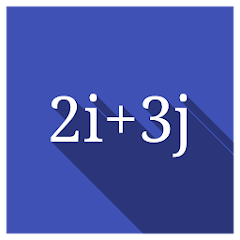# Vectors in 3D Pure Math

1K+Everyone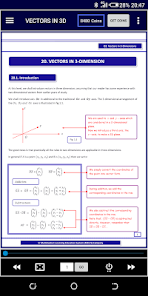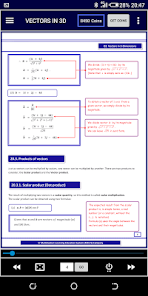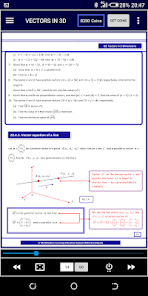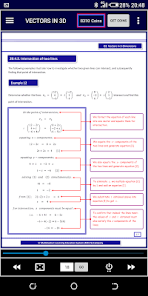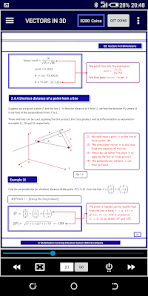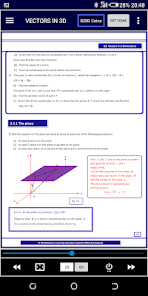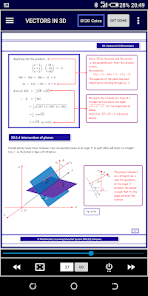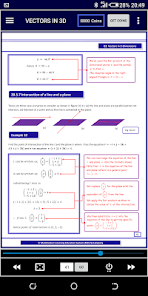Featured sub-topics:
- The Unit vector
- Scalar (dot) and Vector (cross) products
- Vector equation of a line
- Intersection of two lines
- Angle between two lines
- Shortest distance to a line
- Equation of a plane
- Area of a Triangle on a plane
- Area of a Parallelogram on a plane
- Intersection and Angle between planes
- Shortest distance to a plane

Simplified explanations, plus extra side notes with even more explanation!

Over 30 examples per chapter with step by step working.

Past paper examination questions at the end of each chapter.

Check out more Pure Math Chapters here:
Updated on
Sep 19, 2021

## Data safety

Safety starts with understanding how developers collect and share your data. Data privacy and security practices may vary based on your use, region, and age. The developer provided this information and may update it over time.This app may share these data types with third parties
App activity, App info and performance, and Device or other IDsNo data collected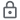Data is encrypted in transit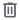You can request that data be deleted

## What's new

All-new user interface with Auto-hiding Toolbars and Page-Flip animation!
TEACH YOURSELF MATH 100% FREE
✸ The Unit vector
✸ Scalar (dot) and Vector (cross) products
✸ Vector equation of a line
✸ Intersection of two lines
✸ Angle between two lines
✸ Shortest distance to a line
✸ Equation of a plane
✸ Area of a Triangle on a plane
✸ Area of a Parallelogram on a plane
✸ Intersection and Angle between planes
✸Shortest distance to a plane